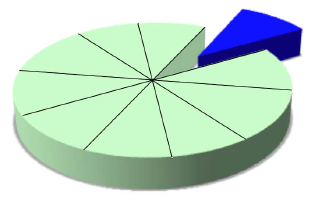# Statistical Learning - Two-fold validation

Two-fold validation is a resampling method. It randomly divides the available set of samples into two parts:

• a training set
• and a validation or hold-out set.

The model is fit on the training set, and the consequent fitted model is used to predict the responses for the observations in the validation set in order to provides an estimate of the test error.

This is typically assessed using:

A random splitting into two halves:

• left part is training set,
• right part is validation set

This is simply a one-stage process. We divide the data set in half, train on one half and predict on the other half.

## Example

Validation is a process that must help us:

In this example, we're comparing the linear model to high-order polynomials in regression.

### One Split

The red curve shows the mean squared error as a function of the degree of the polynomial.

The minimum seems to occur maybe around 2 because it's pretty flat after it. It looks like a quadratic model is probably the best.

### Multiple Splits

When we repeat this process with more and more splits at random into two parts, we get a lot of variability. The minimum does tend to occur around 2 generally but the error is varying from about 16 up to 24, depending on the split. When you divide data in two, you get a lot of variability depending on the split. The shape of the curves are much the same but the actual level of the curve is varying a lot.

### Why so much variability ?

This method is highly variable because we're splitting into two parts.

And because we're splitting in two, we're losing a lot of the (power|information) of the original set. We're throwing away half the data each time in training. We actually want the test error for a training set of size n but we're getting an idea of test error for a training set of size n/2.

And that's likely to be quite a bit higher than the error for a training set of size n.

In general, because the more data,, the more information you have, the lower the error is.

That's why it's a little wasteful if you've got a very small data set. cross-validation will remove that waste and be more efficient.

Recommended Pages(Statistics|Data Mining) - (K-Fold) Cross-validation (rotation estimation)

Cross-validation, sometimes called rotation estimation is a resampling validation technique for assessing how the results of a statistical analysis will generalize to an independent new data set. This...Model Building - ReSampling Validation

Resampling method are a class of methods that estimate the test error by holding out a subset of the training set from the fitting process, and then applying the statistical learning method to those held...